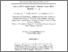# Successful cryptanalytic attacks upon RSA moduli N = pq

## Citation

Abubakar, Saidu Isah and Kamel Ariffin, Muhammad Rezal and Asbullah, Muhammad Asyraf (2019) Successful cryptanalytic attacks upon RSA moduli N = pq. Malaysian Journal of Mathematical Sciences, 13 (spec. Aug.). pp. 141-189. ISSN 1823-8343; ESSN: 2289-750X

## Abstract

This paper reports four new cryptanalytic attacks which show that t instances of RSA moduli Ns = psqs for s = 1, . . . , t where t ≥ 2 can be simultaneously factored in polynomial time using simultaneous Diophantine approximations and lattice basis reduction techniques. We construct four system of equations of the form esd − ksφ(Ns) = 1, esds − kφ(Ns) = 1, esd − kφ(Ns) = zs and esds − kφ(Ns) = zs using N – [(a i+1/i + b i+1/i / 2(ab) i+1/2i + a 1/j + b 1/j / 2(ab) 1/2j) √N] + 1 as a good approximations of φ(Ns) for unknown positive integers d, ds, ks, k, and zs . In our attacks, we found an improved short decryption exponent bound of some reported attacks.Preview
Text
10.pdfView Item